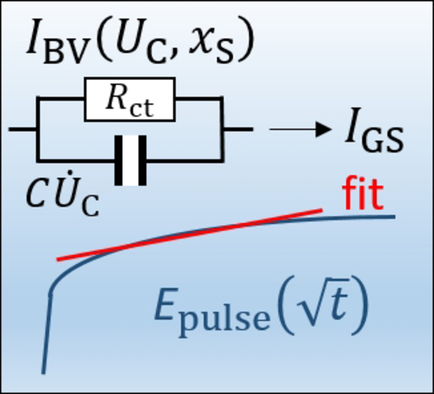Determining the Diffusion Coefficient of Lithium Insertion Cathodes from GITT measurements: Theoretical Analysis for low Temperatures
ChemPhysChem 22, 885 (2021).
T. Schied, A. Nickol, C. Heubner, M. Schneider, A. Michaelis, M. Bobeth, and G. Cuniberti.
https://doi.org/10.1002/cphc.202001025

Accurate knowledge of transport properties of Li-insertion materials in application-relevant temperature ranges is of crucial importance for the targeted optimization of Li-ion batteries (LIBs). Galvanostatic intermittent titration technique (GITT) is a widely applied method to determine Li-ion diffusion coefficients of electrode materials. The well-known calculation formulas based on Weppner's and Huggins’ approach, imply a square-root time dependence of the potential during a GITT pulse. Charging the electrochemical double layer capacitance at the beginning of a GITT pulse usually takes less than one second. However, at lower temperatures down to −40 °C, the double layer charging time strongly increases due to an increase of the charge transfer resistance. The charging time can become comparable with the pulse duration, impeding the conventional GITT diffusion analysis. We propose a model to describe the potential change during a galvanostatic current pulse, which includes an initial, relatively long-lasting double layer charging, and analyze the accuracy of the lithium diffusion coefficient, derived by using the Weppner-Huggins method within a suitably chosen time interval of the pulse. Effects leading to an inaccurate determination of the diffusion coefficient are discussed and suggestions to improve GITT analyses at low temperature are derived.Share

Involved ScientistsDetermining the Diffusion Coefficient of Lithium Insertion Cathodes from GITT measurements: Theoretical Analysis for low Temperatures
ChemPhysChem 22, 885 (2021).
T. Schied, A. Nickol, C. Heubner, M. Schneider, A. Michaelis, M. Bobeth, and G. Cuniberti.
https://doi.org/10.1002/cphc.202001025

Accurate knowledge of transport properties of Li-insertion materials in application-relevant temperature ranges is of crucial importance for the targeted optimization of Li-ion batteries (LIBs). Galvanostatic intermittent titration technique (GITT) is a widely applied method to determine Li-ion diffusion coefficients of electrode materials. The well-known calculation formulas based on Weppner's and Huggins’ approach, imply a square-root time dependence of the potential during a GITT pulse. Charging the electrochemical double layer capacitance at the beginning of a GITT pulse usually takes less than one second. However, at lower temperatures down to −40 °C, the double layer charging time strongly increases due to an increase of the charge transfer resistance. The charging time can become comparable with the pulse duration, impeding the conventional GITT diffusion analysis. We propose a model to describe the potential change during a galvanostatic current pulse, which includes an initial, relatively long-lasting double layer charging, and analyze the accuracy of the lithium diffusion coefficient, derived by using the Weppner-Huggins method within a suitably chosen time interval of the pulse. Effects leading to an inaccurate determination of the diffusion coefficient are discussed and suggestions to improve GITT analyses at low temperature are derived.Share

Involved Scientists